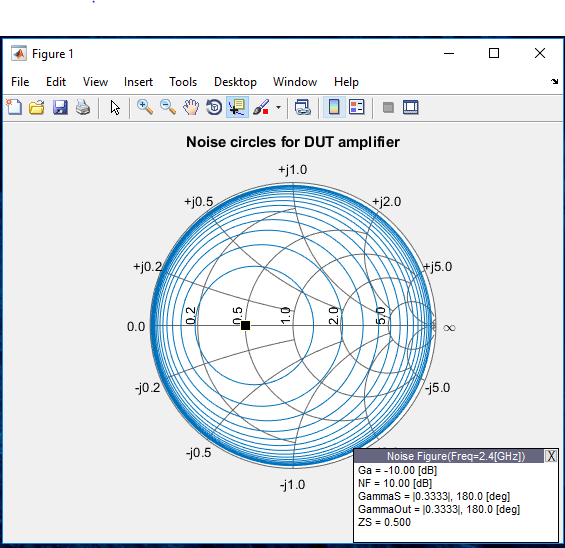Spot Noise Data in Amplifiers and Effects on Measured Noise Figure

This example shows a test bench model to describe the noise introduced by a 2-port device.

The spot noise data parameters, Fmin,opt, and Rn, fully describe the noise introduced by a 2-port device. These parameters along with the source impedance, Zs uniquely determine the measured noise figure of the device. You can use noise circles plotted on a Smith chart to show interaction between Zs and the noise figure.

Measure Noise Figure in RF Blockset

The model Noise_figure_ex simulates a simple noise figure measurement. In this model, the device under test comprises of a single amplifier. To open the model,

open_system('Noise_figure_ex.slx')Click on the Open Script button before running the model. To run the model, select Simulation > Run.

You see that the displayed value settles down at 10.0 dB of the measured noise figure. In this testbench, the amplifier parameters represent a simple attenuator of 10 dB matched to 25 Ohms at both input and output. Due to thermal equilibrium, the expected noise figure of the attenuator is 10 dB when matched, corresponding to the measured value. To gain a broader view of the expected noise figure values for the amplifier when the source impedance deviates from the matched value of 25 Ohm, double-click the Expected Noise Circles subsystem placed in the vicinity of the amplifier:Click Plot noise circles to bring up a figure showing a Smith chart with circles corresponding to the noise figure values specified above the button:The values shown in the Smith chart represent the expected noise figures obtained theoretically from the parameters specified in the amplifier . The Smith chart is interactive and you can place the data cursor on any circle to view the corresponding noise figure, source impedance (normalized to the reference impedance of the amplifier, Z0), and other RF properties. The initial data cursor position corresponds to a point on a noise circle that is closest to the source impedance specified. To control the complex value of this source impedance, double-click the Specify Source Impedance subsystem, and specify the desired value in the edit box.

To validate that the simulated measured noise figure corresponds to theoretical values, specify a reference impedance from the Smith chart, using the Specify Source Impedance subsystem. Run the model again.

Measuring Other RF Systems

You can replace the amplifier in the model by any other RF Blockset system and measure its noise figure. In case the system is frequency depended, you can change the frequency for the measurements by double-clicking the Specify Frequency subsystem and specifying the desired frequency. This frequency is also used for the amplifier noise circles plot.

The plotted expected noise circles apply to the Amplifier block alone. The plotted circles capture correctly all types of data inputs specified in the amplifier, including s2p based network and noise data. Note that the Available Gain shown in the data window of the Smith chart is based on the original data and ignores inaccuracies introduced by the amplifier modeling method. The plotting fails if a block named Amplifier does not exist in the model.

Using Other RF Blockset Blocks

Three additional ways to implement the attenuator specified in the amplifier are:

1. Use an RF Blockset Attenuator block with attenuation of 10dB, with input and output impedances set to 25 Ohms.

2. Implement the attenuator using three resistors arranged in a T ortopology.

3. Use an S-parameter block with the same Scattering matrix used in the amplifier. Select Simulate noise in the block. Simulating noise in a passive S-parameter block accounts for the resistive noise introduced by the S-parameters.